Practice questions available in McGraw Hill Math Grade 2 Answer Key PDF Chapter 6 Test will engage students and is a great way of informal assessment.

Write the time. Write A.M. or P.M.

Morning

Question 1.8:25 A.M.

Explanation:
The hours from midnight to noon are labeled A.M.
If it is 8:25 in the morning, the time is 8:25 A.M.

Afternoon

Question 2.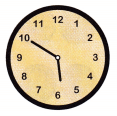5:50 P.M.

Explanation:
The hours from noon to midnight are labeled P.M.
If it is 5:50 in the afternoon, the time is 5:50 P.M.

Look at the clock. Write the time on the digital clock. Then write the time with A.M. or P.M.

Morning

Question 3.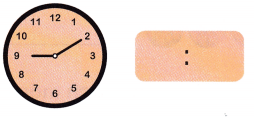Explanation:
The clock shows the time 09:10
The hours from midnight to noon are labeled A.M.
If it is 9:10 in the morning, the time is 9:10 A.M.

Afternoon

Question 4.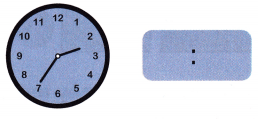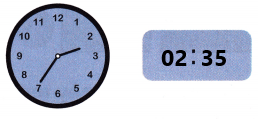Explanation:
The clock shows the time 02:35
The hours from noon to midnight are labeled P.M.
If it is 02:35 in the afternoon, the time is 02:35 P.M.

Count the pennies, nickels, or dimes. Write how much they are worth. Use the ¢ symbol.

Question 5.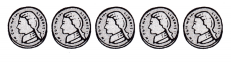30¢

Explanation:
There are 6 nickels
1 nickel = 5 cents
I counted by 5’s
5, 10, 15, 20, 25, 30
So, the coins are worth 30¢.

Question 6.Explanation:
There are 9 pennies
1 penny = 1 cent
I counted by 1’s
So, the coins are worth 9¢.

Question 7.70¢

Explanation:
There are 7 dimes
1 dime = 10 cents
I counted by 10’s
So, the coins are worth 70¢.

Count the dimes, nickels, and pennies by 10s, 5s, and 1s. Write the total. Use the ¢ symbol.

Question 8.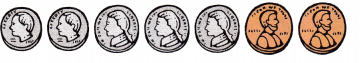37¢

Explanation:
There are 2 dimes
I counted by 10 two times = 20¢
There are 3 nickels
I counted by 5’s 3 times = 15¢
There are 2 pennies
I counted by 1’s= 2¢
20 + 15 + 2 = 37¢
So, the total is 37¢.

Question 9.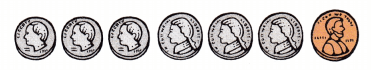46¢

Explanation:
There are 3 dimes
I counted by 10 three times = 30¢
There are 3 nickels
I counted by 5’s 3 times = 15¢
There are 1 penny
I counted by 1’s= 1¢
30 + 15 + 1 = 46¢
So, the total is 46¢.

Count the money. Write the total. Use the ¢ or $symbol. Question 10.Answer: 1$ 75¢

Explanation:
There is 1$There are 3 quarters 1 quarter = 25 cents 2 quarters = 3 x 25 = 75¢ So, the total is 1$ 75¢.

Question 11.75¢

Explanation:
There are 1 quarter
2 quarters = 25¢
There are 3 dimes
2 dimes = 3 x 10 = 30¢
There are 3 nickels
3 nickels = 3 x 5 = 15¢
25+ 30 + 15 = 75¢
So, the total is 75¢.

Question 12.$2.19 Explanation: There is 2 dollars 1 dime = 10¢ 1 nickel = 5¢ 4 pennies = 4¢ 10 + 5 + 4 = 19 So, the total is 1$19¢.

Write how much money the person has. Write how much money the person needs. Use the ¢ or $symbol. Then answer the question. Write yes or no. Question 13. One red marker costs 55¢. Hal has one quarter, two dimes, and two nickels. Hal has ___________. Hal needs ___________. Can he buy a red marker? ____________ Answer: Hal has 55¢. Hal needs 55¢. Can he buy a red marker? Yes Explanation: One red marker costs 55¢ Hal has one quarter, two dimes, and two nickels. 1 quarter = 25¢ 2 dimes = 20¢ 2 nickels = 10¢ 25 + 20 + 10 = 55¢ So, Hal has 55¢ and he can buy one red marker. Question 14. Doug wants to buy a note pad for$1.19. He has a dollar bill, two nickels, and four pennies.
Doug has _____________. Doug needs _____________.
Does he have enough money? ______________
Doug has $1.14. Doug needs$1.19.
Doug wants to buy a note pad for $1.19 He has a dollar bill, two nickels, and four pennies 2 nickels = 10¢ 4 pennies = 4¢ 10 + 4 = 14¢ Doug has$1.14 and he need \$1.19